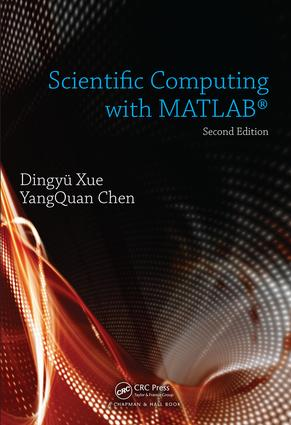# Scientific Computing with MATLAB

## 2nd Edition

Chapman and Hall/CRC

586 pages | 259 B/W Illus.

##### Purchasing Options:\$ = USD
Hardback: 9781498757775
pub: 2016-03-01
SAVE ~\$22.00
\$110.00
\$88.00
x
eBook (VitalSource) : 9781315367859
pub: 2018-09-03
from \$55.00

FREE Standard Shipping!

### Description

Scientific Computing with MATLAB®, Second Edition improves students’ ability to tackle mathematical problems. It helps students understand the mathematical background and find reliable and accurate solutions to mathematical problems with the use of MATLAB, avoiding the tedious and complex technical details of mathematics. This edition retains the structure of its predecessor while expanding and updating the content of each chapter.

The book bridges the gap between problems and solutions through well-grouped topics and clear MATLAB example scripts and reproducible MATLAB-generated plots. Students can effortlessly experiment with the scripts for a deep, hands-on exploration. Each chapter also includes a set of problems to strengthen understanding of the material.

### Reviews

Praise for the First Edition:

"… this volume has many interesting cases to illustrate the power of MATLAB."

Bulletin of the Belgian Mathematical Society, Volume 20, 2013

"The reader’s ability to tackle mathematical problems can be significantly enhanced after reading this book. … This book can be used as a reference text for almost all college students, both undergraduates and graduates, in almost all disciplines that require certain levels of applied mathematics. This book will be a good desktop reference for many who have graduated from college and are still involved in solving mathematical problems in their jobs. A lot of MATLAB function designed by the authors and some third-party free toolboxes are also presented in the book."

Zentralblatt MATH, 1156

Computer Mathematics Languages — An Overview

Computer Solutions to Mathematics Problems

Summary of Computer Mathematics Languages

Outline of the Book

Fundamentals of MATLAB Programming and Scientific Visualization

Essentials in MATLAB Programming

Fundamental Mathematical Calculations

Flow Control Structures of MATLAB Language

Writing and Debugging MATLAB Functions

Two-Dimensional Graphics

Three-Dimensional Graphics

Four-Dimensional Visualization

Calculus Problems

Analytical Solutions to Limit Problems

Analytical Solutions to Derivative Problems

Analytical Solutions to Integral Problems

Series Expansions and Finite-Term Series Approximations

Infinite Series and Products

Path Integrals and Line Integrals

Surface Integrals

Numerical Differentiation

Numerical Integration Problems

Linear Algebra Problems

Inputting Special Matrices

Fundamental Matrix Operations

Fundamental Matrix Transformations

Solving Matrix Equations

Nonlinear Functions and Matrix Function Evaluations

Integral Transforms and Complex-Valued Functions

Laplace Transforms and Their Inverses

Fourier Transforms and Their Inverses

Other Integral Transforms

z Transforms and Their Inverses

Essentials of Complex-Valued Functions

Solving Complex-Valued Function Problems

Solutions of Difference Equations

Nonlinear Equations and Numerical Optimization Problems

Nonlinear Algebraic Equations

Nonlinear Equations with Multiple Solutions

Unconstrained Optimization Problems

Constrained Optimization Problems

Mixed Integer Programming Problems

Linear Matrix Inequalities

Solutions of Multi-Objective Programming Problems

Dynamic Programming and Shortest Path Planning

Differential Equation Problems

Analytical Solution Methods for Some Ordinary Differential Equations

Numerical Solutions to Ordinary Differential Equations

Transforms to Standard Differential Equations

Solutions to Special Ordinary Differential Equations

Solutions to Delay Differential Equations

Solving Boundary Value Problems

Introduction to Partial Differential Equations

Solving ODEs with Block Diagrams in Simulink

Data Interpolation and Functional Approximation Problems

Interpolation and Data Fitting

Spline Interpolation and Numerical Calculus

Fitting Mathematical Models from Data

Rational Function Approximations

Special Functions and Their Plots

Signal Analysis and Digital Signal Processing

Probability and Mathematical Statistics Problems

Probability Distributions and Pseudorandom Numbers

Solving Probability Problems

Fundamental Statistical Analysis

Statistical Estimations

Statistical Hypothesis Tests

Analysis of Variance

Principal Component Analysis

Topics on Nontraditional Mathematical Branches

Fuzzy Logic and Fuzzy Inference

Rough Set Theory and Its Applications

Neural Network and Applications in Data Fitting Problems

Evolutionary Computing and Global Optimization Problem Solutions

Wavelet Transform and Its Applications in Data Processing

Fractional-Order Calculus

MATLAB Functions Index

Index

Exercises and Bibliography appear at the end of each chapter.

### About the Authors

Dingyü Xue is a professor of control engineering in the College of Information Science and Engineering at Northeastern University in China. Dr. Xue is the author or coauthor of many books on MATLAB®-based control, simulation, and mathematical problem solutions, including Computer Aided Control Systems Design with MATLAB, Solving Advanced Applied Mathematical Problems Using MATLAB, and System Simulation Techniques with MATLAB and Simulink. His current research interests include CAD of control systems, system simulation, and fractional order control. He earned his D.Phil. from Sussex University.

YangQuan Chen is the director and founder of the Mechatronics, Embedded Systems and Automation (MESA) Lab in the School of Engineering at the University of California, Merced. He is the topic editor-in-chief of field robotics for the International Journal of Advanced Robotic Systems, a founding editorial board member of Unmanned Systems, and an editorial board member of several other leading journals. Dr. Chen is also the author or coauthor of many books, including Distributed-Order Dynamic Systems: Stability, Simulation, Applications and Perspectives, Fractional Order Motion Controls, Remote Sensing and Actuation Using Unmanned Vehicles, System Simulation Techniques with MATLAB and Simulink, and Modeling, Analysis and Design of Control Systems in MATLAB and Simulink. His research interests include unmanned aerial systems and UAV-based personal remote sensing, cyber-physical systems, the modeling and control of renewable energy systems, mechatronics, and applied fractional calculus. He earned his Ph.D. from Nanyang Technological University.

### Subject Categories

##### BISAC Subject Codes/Headings:
MAT003000
MATHEMATICS / Applied
MAT021000
MATHEMATICS / Number Systems